# Students

In the front row sitting three students and in every other row 11 students more than the previous row. Determine how many students are in the room when the room is 9 lines, and determine how many students are in the seventh row.

Correct result:

x =  423
a7 =  69

#### Solution:

$a=3 \ \\ d=11 \ \\ n=9 \ \\ b=a+(n-1) \cdot \ d=3+(9-1) \cdot \ 11=91 \ \\ x=n \cdot \ (a+b)/2=9 \cdot \ (3+91)/2=423$
$a_{7}=a+(7-1) \cdot \ d=3+(7-1) \cdot \ 11=69$We would be pleased if you find an error in the word problem, spelling mistakes, or inaccuracies and send it to us. Thank you!## Next similar math problems:

• Chairs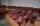Determine the number of seats in the seventh row and ninth row, if 3rd row has 14 seats and in every next row of seats has five more than the previous row.
• Eight palm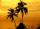There grows 8 palms by the sea. At the first sitting one parrot, on second two, on third sits four parrots on each other twice the previous parrots sitting on a previous palm. How many parrots sitting on eighth palm?
• Cans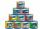How many cans must be put in the bottom row if we want 182 cans arrange in 13 rows above so that each subsequent row has always been one tin less? How many cans will be in the top row?
• Cinema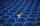How many rows of seats are in the stands, if you know that the first row has 24 seats and the last 50 seats and each subsequent row has two seats more than row before? How many seats are in the stands?
• LinesIn how many points will intersect 14 different lines, where no two are parallel?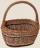Determine how many apples are in baskets when in the first basket are 4 apples, and in any other is 29 apples more than the previous, and we have eight baskets.
• Sheep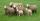Shepherd tending the sheep. Tourists asked him how much they have. The shepherd said, "there are fewer than 500. If I them lined up in 4-row 3 remain. If in 5-row 4 remain. If in 6-row 5 remain. But I can form 7-row." How many sheep have herdsman?
• Ravens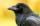The tale of the Seven Ravens were seven brothers, each of whom was born exactly 2.5 years after the previous one. When the eldest of the brothers was 2-times older than the youngest, mother all curse. How old was seven ravens brothers when their mother cu
• Three ints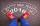The sum of three consecutive integers is 2016. What numbers are they?
• Roof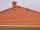Tiles are stacked in rows on the trapezoidal shaped roof. At the ridge is 15 tiles and each subsequent row has one more tile than in the previous row. How many tiled is covered roof if lowermost row has 37 tiles?
• Table 3x3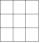Table with numbers 3x3 in the first row 66,24,33 in the second row 57,? 19 in the third row 18,45,60 What number comes ar mark ?. Maybe the numbers: 22,46,45,47
• Consecutive numbersSum of ten consecutive numbers is 105. Determine these numbers (write first and last).
• Salat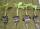Grandmother planted salad. In each row he planted 13 seedlings. After a morning frost many seedlings died. The first row five seedlings died. In the second row two seedlings died more than 1st row. In the third row three seedlings died less than 1st row.
• Series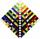Your task is express the sum of the following arithmetic series for n = 14: S(n) = 11 + 13 + 15 + 17 + ... + 2n+9 + 2n+11
• Chocholate pyramid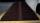How many chocolates are in the third shelf when at the 8th shelf are 41 chocolates in any other shelf is 7 chocolates more the previous shelf.
• NumbersDetermine the number of all positive integers less than 4183444 if each is divisible by 29, 7, 17. What is its sum?
• The HotelThe Holiday Hotel has the same number of rooms on each floor. Rooms are numbered with natural numerals sequentially from the first floor, no number is omitted, and each room has a different number. Three tourists arrived at the hotel. The first one was in